• Save

Profit Loss Formulas For Competitive Exams

8 months ago 853 Views
NEW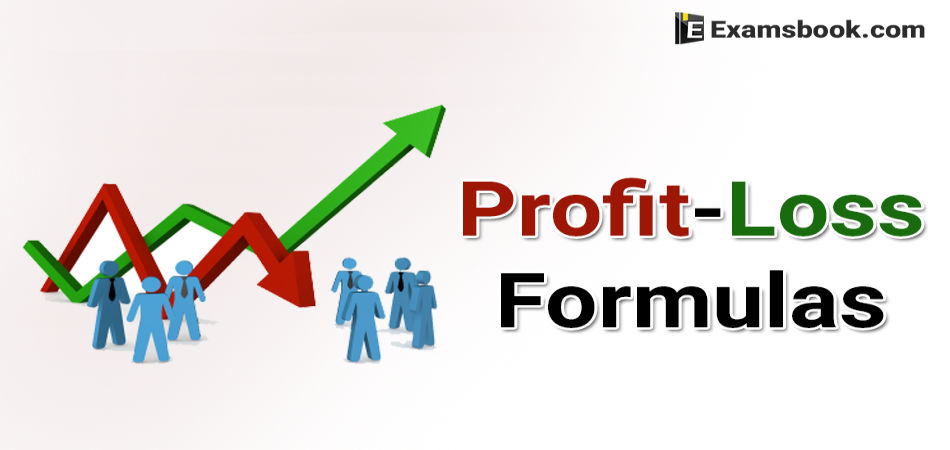Profit-Loss is an important topic which questions asks in the competitive exams. Profit and loss questions are based on transaction. So, here I am telling you about profit and loss formulas that how to use formulas while solving these questions.

Here are solved profit-loss questions so that you will be able to understand these questions. Instead of that, here are also given questions with solutions for your better practice or preparation.

You can easily solve these type of questions if you know Profit and Loss Trick to solve. Click on Profit-Loss Formulas to know more formulas.

Formulas of Profit and Loss

Cost Price (CP)

The money paid by the shopkeeper to the manufacturer or money paid by the customer to the shopkeeper is called the cost price (CP) of the goods.

Selling Price (SP)

The price at which the shopkeeper sells the goods is called the selling price (SP).

Profit or Gain

Whenever, a person sells an article at price greater than the cost price, he is said to have made a profit or gain.

Profit or Gain = SP – CP [SP>CP]

Loss

If the selling price of an article is less than its cost price, then the shopkeeper suffers a loss.

Loss = CP – SP [CP>SP]

Example of Profit loss formula with question.

1. If a person sells two similar articles, one at a gain of a% and another at a loss of a%, then the seller always incurs a loss which is given by loss percent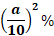A man sold two radios for Rs. 2000 each. On one he gains 16% and on the other he losses 16%. Find his gain or loss percent in the whole transaction.

(A) 4.00

(B) 2.56

(C) 3.36

(D) 2.50

According to the formula,

Loss percent = (a/10)2= (16/10)2= 256/100 = 2.56%.

2. If a dishonest trader professes to sell his items at CP but uses false weight, then

Or

A dishonest dealer professes to sell his goods at cost price but he uses a weight of 930 g for a kg weight. Find his gain percent.

(A)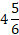(B)(C)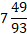(D) 0

According to the formula,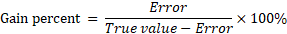3. If cost price of a articles is equal to the selling of b articles, then profit percentage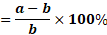If the cost price of 20 articles is equal to the selling price of 18 articles, find the profit per cent.

(A)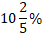(B)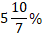(C)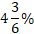(D)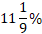Given that, a = 20, b = 18

According to the formula,

4. A tradesman marks his goods at a% above the cost price and gives purchases at discount of b% for cash, there is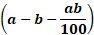% profit or loss according to (+) ve or (-) ve sign.

A tradesman marks his goods at 20% above the cost price and allows purchase a discount of 10% for cash. What profit percent does he make?

(A) 6

(B) 0

(C) 8

(D) 10

Given that, a = 20%, b = 10%

According to the formula, Required answer =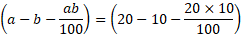= (20-12) = 8% Profit.

5. A businessman sells his items at a profit a%. If he had sold it for Rs R more, he would have gained b% Then,

A person sold a table at profit of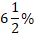. If he had sold it for Rs. 1250 more, he would have gained 19%. Find the CP of the table.

(A) Rs. 10000

(B) Rs. 20000

(C) Rs. 15300

(D) Rs. 2000

Here,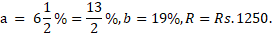According to the formula,

Practice Questions and Answers

Q.1. A person sold two cows each for Rs. 9900. If he gained 10% on one and lost 20% on the other, then which of the following is true?

(A) He gained Rs. 200

(B) He lost Rs. 200

(C) He neither gained nor lost

(D) None of these

Ans .   D

The CP of profitable cow

= 9900/1.1 = Rs. 9000

And profit = Rs. 900

The CP of loss yielding cow = 9900/0.8

= Rs. 12375 and loss = Rs. 2475

So, the net loss

= 2475 – 900 = Rs. 1575.

Q.2. A shopkeeper sold 12 cameras at a profit of 20% and 8 camera at a profit 10%. If he had sold all the 20 cameras at a profit of 15%, then his profit would have been reduced by Rs. 36. What is the cost price of each camera?

(A) Rs. 100

(B) Rs. 150

(C) Rs. 180

(D) Rs. 220

Ans .   C

Go through option

180×12×1.2+180×8×1.1

= 180[14.4+8.8]

= 180(23.2) = 4176.

And 180×20×11.5=4140

There fore loss = 4176 – 4140 = 36.

Hence, option (C) is correct.

Q.3. A man by 400 orange at Rs. 4 and 500 orange at Rs. 2. He mixes them together and sells them at Rs. 3. Find his percent loss or gain.

(A)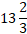(B)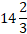(C)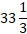(D)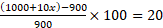20

Ans .  B

Total CP =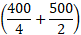= Rs. 350.

Total SP =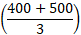= Rs. 300

Percent profit =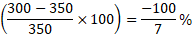⸫ Percent loss =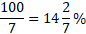Q.4. A man sells two articles, each for the same price of Rs. 420. He earns 20% profit on the first and 40% profit on the second. Find his overall percent profit.

(A) 27

(B) 33.3

(C) 37.25

(D) 29.23

Ans .   D

SP of each article = Rs. 420

Total SP of both articles = Rs. 840

CP of first article =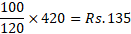CP of Second article =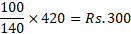Total CP of both articles = (350+300) = Rs. 650

Overall per cent gain

Q.5. A balance of a trader weighs 10% less than it should be. Still the trader marks up his goods to get the overall profit of 20%. What is the marks up on the cost price?

(A) 40%

(B) 8%

(C) 25%

(D) 16.66%

Ans .   B

Let the CP be Rs 1 per g,

But he weighs 900 g for every 1000 g.

⸫ Value of goods sold = 900

Now, let the marks up be x%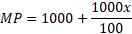= (1000+10x)

But, Since MP = SP

⸫ SP = (1000+10x)

Hence, Profit percent

=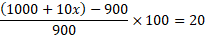⸫ X = 8.

Ask me anything in the comment section if you face any problem in profit-loss fromulas.